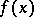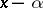# Bezout theorem

(diff) ← Older revision | Latest revision (diff) | Newer revision → (diff)
Jump to: navigation, search

Bezout's theorem on the division of a polynomial by a linear binomial: The remainder of the division of the polynomialby the binomialis. It is assumed that the coefficients of the polynomials are contained in a certain commutative ring with a unit element, e.g. in the field of real or complex numbers. A consequence of Bezout's theorem is the following: A numberis a root of the polynomialif and only ifis divisible by the binomialwithout remainder.

Bezout's theorem on homogeneous equations: If a system ofhomogeneous equations inunknowns(*)

has only a finite number of non-proportional non-zero solutions in an algebraically closed field containing the coefficients of the system, then the number of these solutions counted according to their multiplicity is equal to the product of the degrees of the equations. The multiplicity of the solutions is, by definition, the intersection index (in algebraic geometry) of the hypersurfaces (*) at the respective point. The theorem is called after E. Bezout , who studied systems of algebraic equations of higher degrees.

How to Cite This Entry:
Bezout theorem. Encyclopedia of Mathematics. URL: http://encyclopediaofmath.org/index.php?title=Bezout_theorem&oldid=11972
This article was adapted from an original article by V.N. RemeslennikovV.E. Voskresenskii (originator), which appeared in Encyclopedia of Mathematics - ISBN 1402006098. See original article